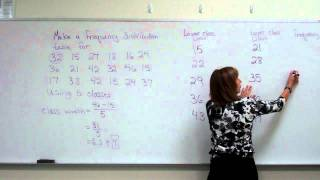#### Making A Frequency Distribution Table

##### Transcript:

[2.689]

[430.959]
and on the other side of the upper-class limits will write upper-class father it's just another way to denote your classes pay the boundaries our way they meet so look at where this first class ends at 21 and the second class starts at 22 those bars if you graph them on graph would not touch and we want to have this information where we might be able to use it for a histogram so we want to have where the bars would touch so we're going to share the distance between 21 and 22 which would mean that we would end this first class at 21.5 and as a upper class boundary and start the second classes lower class boundary at all so that 21.5 so we're meeting halfway for that point of our lower class boundary in our upper class boundary so for the second class it will go up to twenty-eight point five and twenty eight point five is the lower class boundary of the third class thirty five point five is the upper class boundary of the third class and so that would give us thirty five point five as the lower class boundary of the fourth class and then for the fourth classes upper class boundary about forty two point five and that's the lower class boundary of the fifth class and then the last thing we need to do is make sure that the spans are all equally wimped so we would need to go down a half on our first entry of our first class so this will have a lower class boundary 14.5 and the last class will have an upper quest found tree of forty nine point five so another way to denote your classes the last thing I want to do for this segment is to also get some information that we would need if we are looking at trying to use the information off the frequency distribution table to graph a frequency polygon and with the frequency polygon we want to find what the midpoints are of each class and the midpoints we just take and add the two class limits the lower class women and the upper class limit divided by 215 plus 21 is 36 and 36 divided by 2 is 18 you could have also have added the class boundaries of the class and divided by 2 and you would get that same number 18 now we can do that for each class however your class midpoints are also going to be the class width apart so once you have your first class midpoint you can find the rest of them by just adding the cuts width to it to get the rest of them so 18 plus 7 is twenty five twenty five point seven is thirty-two thirty-two plus seven is 39 and 39 plus seven is 46 notice that with each of these if I were to do the other way of the average 22 plus 28 is 50 and 50 divided by 2 is 25 I would still get the same answer 29 plus 35 is going to of me 64 and 64 divided by 2 is from a 32 etc through all of it so here we have ways of getting the information for our frequency distribution table this is a frequency distribution table and working with a frequency distribution table so that we have all the information we need to pull it off and draw histograms and

##### More from this creator:
###### Disclaimer:
TranscriptionTube is a participant in the Amazon Services LLC Associates Program, an affiliate advertising program designed to provide a means for sites to earn advertising fees by advertising and linking to amazon.com
###### Contact:
You may contact the administrative operations team of TranscriptionTube with any inquiries here: Contact